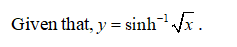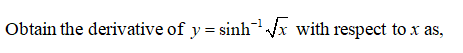# In Exercises 25–27, find the derivative of y with respect to the appro-priate variable.25. y = sinh-1sqrt(x)26. y = cosh-1 2sqrt(x + 1)27. y = (1 - u) tanh-1 u

Question
1 views

In Exercises 25–27, find the derivative of y with respect to the appro-priate variable.

25. y = sinh-1sqrt(x)

26. y = cosh-1 2sqrt(x + 1)

27. y = (1 - u) tanh-1 u

check_circle

Step 1

Hey since there are multiple questions the first question is answered and repost the question if any particular question has to answered.

Step 2Step 3...

### Want to see the full answer?

See Solution

#### Want to see this answer and more?

Solutions are written by subject experts who are available 24/7. Questions are typically answered within 1 hour.*

See Solution
*Response times may vary by subject and question.
Tagged in

### Other